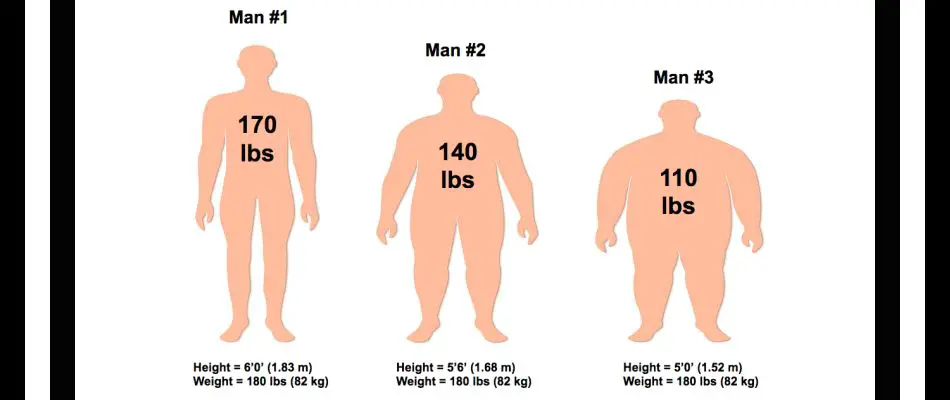# Ideal Body Weight Calculator## Weight for Height Calculation for Men & Women

If you are wondering how much should I weigh, use our Ideal Body Weight Calculator to find our your ideal body weight for your height and built.

Just enter your gender (women / men), height and frame size, and the weight calculator will calculate your ideal body weight for height. The body weight is provided in pounds as well as Kilograms.

For measuring ideal body weight, a measurement that is more commonly used today is body mass index (BMI). The BMI is thought to be a more accurate reflection of total body fat than the comparison of height and weight measurements.

## Ideal Body Weight Calculator

Ideal Weight for Men & Women

Yor height

Ideal body weight in kilograms
Ideal body weight in pounds

Mean
Range
to
to

Automatic recalculation

Also Check: BMI Chart | Ideal Body Weight Calculator | Homeopathic Medicine for Weight Loss | Recommended Calorie Intake Calculator | Calculate Body Fat Percentage | Calories Burned Calculator | Calories Intake Calculator | Weight Loss Calculator

## Ideal Body Weight Calculation

Determining desirable body weight for height for men and women

If the tables are not available, a simple way to determine desirable body weight is as follows:

Women: 100 pounds of body weight for the first 5 feet of height, 5 pounds for each additional inch.
Men: 106 pounds of body weight for the first 5 feet of height, 6 pounds for each additional inch.
Add 10% for a large frame size, and subtract 10% for a small frame size.

Determining frame size to calculate ideal body weight:

To determine the body frame size, measure the wrist with a tape measure and use the following chart to determine whether the person is small, medium, or large boned.

### Women

height under 5'2"

small=wrist size less than 5.5"
medium=wrist size 5.5" to 5.75"
large=wrist size over 5.75"

height 5'2" to 5' 5"

small=wrist size less than 6"
medium=wrist size 6" to 6.25"
large=wrist size over 6.25"

height over 5' 5"

small=wrist size less than 6.25"
medium=wrist size 6.25" to 6.5"
large=wrist size over 6.5"

### Men

height over 5' 5"

small=wrist size 5.5" to 6.5"
medium=wrist size 6.5" to 7.5"
large=wrist size over 7.5"

Using the above formula, you can calculate your desirable ideal body weight for both males and females. The formula provides an average body weight for height and frame. The weight range can vary on an individual basis and between mean and women.

These weight tables are only appropriate to use with adults. For children, the height, weight, and head circumference should be measured and plotted in relationship to age on a growth chart at each well child check from 2 weeks through 3 years of age. After age 3, only the height and weight are measured and recorded. Each individual growth measurement is not that helpful to the pediatrician, but the pattern of growth over time is extremely important.

Average weight to height calculation with Ideal Body Weight Calculator. Calculate ideal weight for men and women.